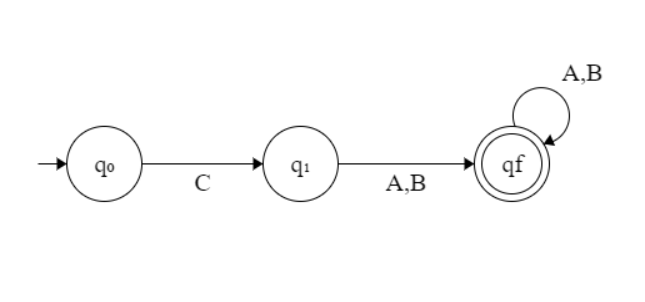Open in App
Not now

# Program to construct DFA for Regular Expression C( A + B)+

• Last Updated : 10 Feb, 2022

Given a string S, the task is to design a Deterministic Finite Automata (DFA) for accepting the language L = C (A + B)+. If the given string is accepted by DFA, then print “Yes”. Otherwise, print “No”.

Examples:

Input: S = “CABABABAB”
Output: Yes
Explanation: The given string is of the form C(A + B)+ as the first character is C and it is followed by A or B.

Input: S = “ABAB”
Output: No

Approach: The idea is to interpret the given language L = C (A + B)+ and for the regular expression of the form C(A + B)+, the following is the DFA State Transition Diagram:Follow the steps below to solve the problem:

• If the given string is of length less than equal to 1, then print “No”.
• If the first character is always C, then traverse the remaining string and check if any of the characters is A or B.
• If there exists any character other than A or B while traversing in the above step, then print “No”.
• Otherwise, print “Yes”.

Below is the implementation of the above approach:

## C++

 `// C++ program for the above approach`   `#include ` `using` `namespace` `std;`   `// Function to find whether the given` `// string is Accepted by the DFA` `void` `DFA(string str, ``int` `N)` `{` `    ``// If n <= 1, then print No` `    ``if` `(N <= 1) {` `        ``cout << ``"No"``;` `        ``return``;` `    ``}`   `    ``// To count the matched characters` `    ``int` `count = 0;`   `    ``// Check if the first character is C` `    ``if` `(str == ``'C'``) {` `        ``count++;`   `        ``// Traverse the rest of string` `        ``for` `(``int` `i = 1; i < N; i++) {`   `            ``// If character is A or B,` `            ``// increment count by 1` `            ``if` `(str[i] == ``'A'` `|| str[i] == ``'B'``)` `                ``count++;` `            ``else` `                ``break``;` `        ``}` `    ``}` `    ``else` `{`   `        ``// If the first character` `        ``// is not C, print -1` `        ``cout << ``"No"``;` `        ``return``;` `    ``}`   `    ``// If all characters matches` `    ``if` `(count == N)` `        ``cout << ``"Yes"``;` `    ``else` `        ``cout << ``"No"``;` `}`   `// Driver Code` `int` `main()` `{` `    ``string str = ``"CAABBAAB"``;` `    ``int` `N = str.size();` `    ``DFA(str, N);`   `    ``return` `0;` `}`

## Java

 `// Java program for the above approach` `import` `java.util.*;` `class` `GFG` `{`   `  ``// Function to find whether the given` `  ``// String is Accepted by the DFA` `  ``static` `void` `DFA(String str, ``int` `N)` `  ``{`   `    ``// If n <= 1, then print No` `    ``if` `(N <= ``1``)` `    ``{` `      ``System.out.print(``"No"``);` `      ``return``;` `    ``}`   `    ``// To count the matched characters` `    ``int` `count = ``0``;`   `    ``// Check if the first character is C` `    ``if` `(str.charAt(``0``) == ``'C'``)` `    ``{` `      ``count++;`   `      ``// Traverse the rest of String` `      ``for` `(``int` `i = ``1``; i < N; i++)` `      ``{`   `        ``// If character is A or B,` `        ``// increment count by 1` `        ``if` `(str.charAt(i) == ``'A'` `|| ` `            ``str.charAt(i) == ``'B'``)` `          ``count++;` `        ``else` `          ``break``;` `      ``}` `    ``}` `    ``else` `    ``{`   `      ``// If the first character` `      ``// is not C, print -1` `      ``System.out.print(``"No"``);` `      ``return``;` `    ``}`   `    ``// If all characters matches` `    ``if` `(count == N)` `      ``System.out.print(``"Yes"``);` `    ``else` `      ``System.out.print(``"No"``);` `  ``}`   `  ``// Driver Code` `  ``public` `static` `void` `main(String[] args)` `  ``{` `    ``String str = ``"CAABBAAB"``;` `    ``int` `N = str.length();` `    ``DFA(str, N);` `  ``}` `}`   `// This code is contributed by 29AjayKumar`

## Python3

 `# Python3 program for the above approach`   `# Function to find whether the given` `# is Accepted by the DFA` `def` `DFA(``str``, N):` `    `  `    ``# If n <= 1, then print No` `    ``if` `(N <``=` `1``):` `        ``print``(``"No"``)` `        ``return`   `    ``# To count the matched characters` `    ``count ``=` `0`   `    ``# Check if the first character is C` `    ``if` `(``str``[``0``] ``=``=` `'C'``):` `        ``count ``+``=` `1`   `        ``# Traverse the rest of string` `        ``for` `i ``in` `range``(``1``, N):`   `            ``# If character is A or B,` `            ``# increment count by 1` `            ``if` `(``str``[i] ``=``=` `'A'` `or` `str``[i] ``=``=` `'B'``):` `                ``count ``+``=` `1` `            ``else``:` `                ``break` `    ``else``:` `        ``# If the first character` `        ``# is not C, print -1` `        ``print``(``"No"``)` `        ``return`   `    ``# If all characters matches` `    ``if` `(count ``=``=` `N):` `        ``print``(``"Yes"``)` `    ``else``:` `        ``print``(``"No"``)`   `# Driver Code` `if` `__name__ ``=``=` `'__main__'``:` `    ``str` `=` `"CAABBAAB"` `    ``N ``=` `len``(``str``)` `    ``DFA(``str``, N)`   `# This code is contributed by mohit kumar 29.`

## C#

 `// C# program for the above approach` `using` `System;` `class` `GFG` `{`   `  ``// Function to find whether the given` `  ``// String is Accepted by the DFA` `  ``static` `void` `DFA(``string` `str, ``int` `N)` `  ``{`   `    ``// If n <= 1, then print No` `    ``if` `(N <= 1)` `    ``{` `      ``Console.Write(``"No"``);` `      ``return``;` `    ``}`   `    ``// To count the matched characters` `    ``int` `count = 0;`   `    ``// Check if the first character is C` `    ``if` `(str == ``'C'``) {` `      ``count++;`   `      ``// Traverse the rest of String` `      ``for` `(``int` `i = 1; i < N; i++) {`   `        ``// If character is A or B,` `        ``// increment count by 1` `        ``if` `(str[i] == ``'A'` `            ``|| str[i] == ``'B'``)` `          ``count++;` `        ``else` `          ``break``;` `      ``}` `    ``}` `    ``else` `{`   `      ``// If the first character` `      ``// is not C, print -1` `      ``Console.Write(``"No"``);` `      ``return``;` `    ``}`   `    ``// If all characters matches` `    ``if` `(count == N)` `      ``Console.Write(``"Yes"``);` `    ``else` `      ``Console.Write(``"No"``);` `  ``}`   `  ``// Driver Code` `  ``static` `public` `void` `Main()` `  ``{`   `    ``string` `str = ``"CAABBAAB"``;` `    ``int` `N = str.Length;` `    ``DFA(str, N);` `  ``}` `}`   `// This code is contributed by Dharanendra L V`

## Javascript

 ``

Output:

`Yes`

Time Complexity: O(N)
Auxiliary Space: O(1)

My Personal Notes arrow_drop_up
Related Articles